1595235900

# Intuitive Understanding of Randomized Singular Value Decomposition

Matrix decomposition is a foundational tool for many machine learning problems and is widely used in data compression, dimensionality reduction, and sparsity learning, to name but a few. In many cases, for purposes of approximating a data matrix by a low-rank structure, Singular Value Decomposition (SVD) is often verified as the best choice. However, the accurate and efficient SVD of large-scale data matrices is computationally challenging. To resolve the SVD in this situation, there are many algorithms have been developed by applying randomized linear algebra. One of the most important algorithms is randomized SVD, which is competitively efficient for decomposing any large matrix with a relatively low rank.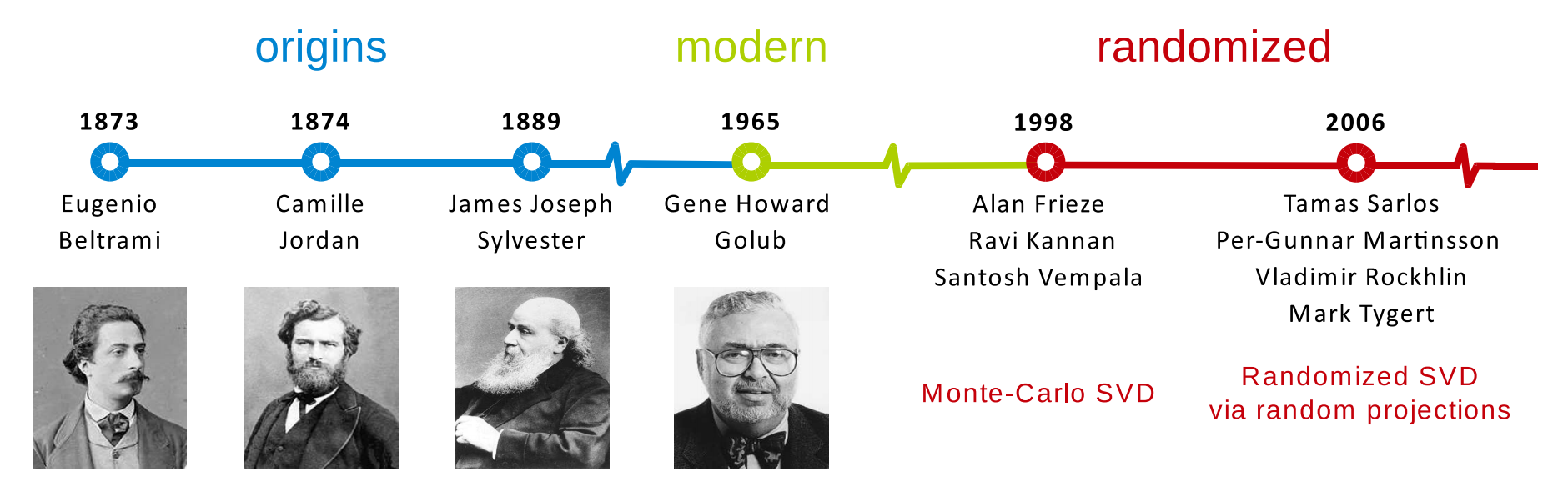Figure 1: A timeline of major SVD developments. (The picture is from )

This post will introduce the preliminary and essential idea of the randomized SVD. To help readers gain a better understanding of randomized SVD, we also provide the corresponding Python implementation in this post. In addition, Jupyter notebook of this post is available at here.

#### Preliminary

SVD Formula

As you may already know, SVD is one of the most important decomposition formula in linear algebra. For any given matrix A, SVD has the form of

A = U_Σ_V^T

where the matrices U and**_ V _**consist of left and right singular vectors, respectively. The diagonal entries of Σ are singular values.

A Small Matrix Example

Take a 3-by-3 matrix for example, we can compute the SVD by using `numpy.linalg.svd()` in Python. Let us have a look:

``````import numpy as np

A = np.array([[1, 3, 2],
[5, 3, 1],
[3, 4, 5]])
u, s, v = np.linalg.svd(A, full_matrices = 0)
print('Left singular vectors:')
print(u)
print()
print('Singular values:')
print(s)
print()
print('Right singular vectors:')
print(v)
print()
``````

In this case, the singular values are 9.3427, 3.2450, and 1.0885.

``````Left singular vectors:
[[-0.37421754 0.28475648 -0.88253894]
[-0.56470638 -0.82485997 -0.02669705]
[-0.7355732 0.48838486 0.46948087]]
Singular values:
[9.34265841 3.24497827 1.08850813]
Right singular vectors:
[[-0.57847229 -0.61642675 -0.53421706]
[-0.73171177 0.10269066 0.67383419]
[ 0.36051032 -0.78068732 0.51045041]]
``````

#linear-algebra #signal-processing #machine-learning #matrix-decomposition #numpy

## Buddha Community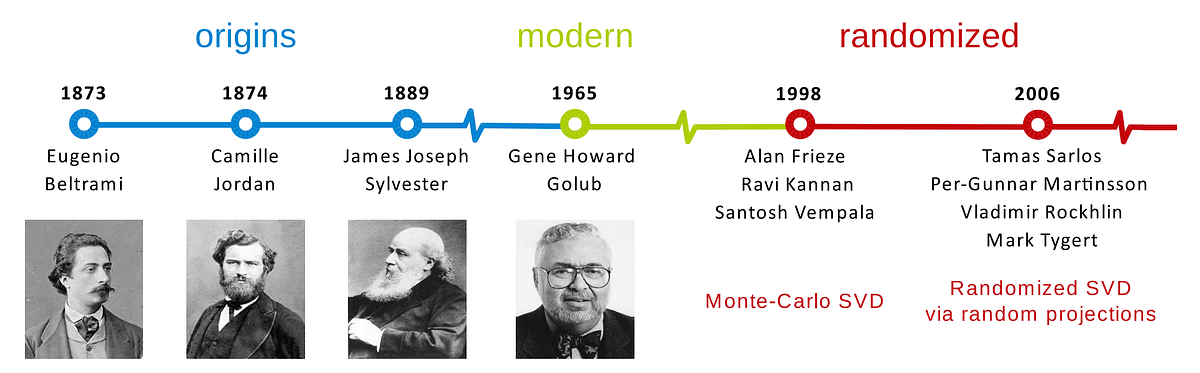1595235900

## Intuitive Understanding of Randomized Singular Value Decomposition

Matrix decomposition is a foundational tool for many machine learning problems and is widely used in data compression, dimensionality reduction, and sparsity learning, to name but a few. In many cases, for purposes of approximating a data matrix by a low-rank structure, Singular Value Decomposition (SVD) is often verified as the best choice. However, the accurate and efficient SVD of large-scale data matrices is computationally challenging. To resolve the SVD in this situation, there are many algorithms have been developed by applying randomized linear algebra. One of the most important algorithms is randomized SVD, which is competitively efficient for decomposing any large matrix with a relatively low rank.Figure 1: A timeline of major SVD developments. (The picture is from )

This post will introduce the preliminary and essential idea of the randomized SVD. To help readers gain a better understanding of randomized SVD, we also provide the corresponding Python implementation in this post. In addition, Jupyter notebook of this post is available at here.

#### Preliminary

SVD Formula

As you may already know, SVD is one of the most important decomposition formula in linear algebra. For any given matrix A, SVD has the form of

A = U_Σ_V^T

where the matrices U and**_ V _**consist of left and right singular vectors, respectively. The diagonal entries of Σ are singular values.

A Small Matrix Example

Take a 3-by-3 matrix for example, we can compute the SVD by using `numpy.linalg.svd()` in Python. Let us have a look:

``````import numpy as np

A = np.array([[1, 3, 2],
[5, 3, 1],
[3, 4, 5]])
u, s, v = np.linalg.svd(A, full_matrices = 0)
print('Left singular vectors:')
print(u)
print()
print('Singular values:')
print(s)
print()
print('Right singular vectors:')
print(v)
print()
``````

In this case, the singular values are 9.3427, 3.2450, and 1.0885.

``````Left singular vectors:
[[-0.37421754 0.28475648 -0.88253894]
[-0.56470638 -0.82485997 -0.02669705]
[-0.7355732 0.48838486 0.46948087]]
Singular values:
[9.34265841 3.24497827 1.08850813]
Right singular vectors:
[[-0.57847229 -0.61642675 -0.53421706]
[-0.73171177 0.10269066 0.67383419]
[ 0.36051032 -0.78068732 0.51045041]]
``````

#linear-algebra #signal-processing #machine-learning #matrix-decomposition #numpy1625013180

## Generate Random Numbers in Python

There are two types of random number generators: pseudo-random number generator and true random number generator.

Pseudorandom numbers depend on computer algorithms. The computer uses algorithms to generate random numbers. These random numbers are not truly random because they are predictable like the generated numbers using NumPy random seed.

Whereas, truly random numbers are generated by measuring truly physical random parameters so we can ensure that the generated numbers are truly random.

The pseudo-random numbers are not safe to use in cryptography because they can be guessed by attackers.

In Python, the built-in random module generates pseudo-random numbers. In this tutorial, we will discuss both types. So let’s get started.

#python #random #generate random numbers #random numbers #generate random numbers in python1597123980

## Recommender System — singular value decomposition (SVD) & truncated SVD

The most common method for recommendation systems often comes with Collaborating Filtering (CF) where it relies on the past user and item dataset. Two popular approaches of CF are latent factor models, which extract features from user and item matrices and neighborhood models, which finds similarities between products or users.

The neighborhood model is an item-oriented approach to discover the user preference based on the ratings given by the user for similar items. On the other hand, latent factor models such as Singular Value Decomposition (SVD) extract features and correlation from the user-item matrix. For example, when items are movies in different categories. SVD would generate factors when looking into the dimension space like action vs comedy, Hollywood vs Bollywood, or Marvel vs Disney. Mainly, we will focus on the latent factor model for the Singular Value Decomposition (SVD) approach.

In this article, you will learn the singular value decomposition and truncated SVD of the recommender system:

(1) Introduction to singular value decomposition

(2) Introduction to truncated SVD

(3) Hands-on experience of python code on matrix factorization

## Introduction to singular value decomposition

When it comes to dimensionality reduction, the Singular Value Decomposition (SVD) is a popular method in linear algebra for matrix factorization in machine learning. Such a method shrinks the space dimension from N-dimension to K-dimension (where K<N) and reduces the number of features. SVD constructs a matrix with the row of users and columns of items and the elements are given by the users’ ratings. Singular value decomposition decomposes a matrix into three other matrices and extracts the factors from the factorization of a high-level (user-item-rating) matrix.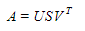Matrix U: singular matrix of (user*latent factors)

Matrix S: diagonal matrix (shows the strength of each latent factor)

Matrix U: singular matrix of (item*latent factors)

From matrix factorization, the latent factors show the characteristics of the items. Finally, the utility matrix A is produced with shape m*n. The final output of the matrix A reduces the dimension through latent factors’ extraction. From the matrix A, it shows the relationships between users and items by mapping the user and item into r-dimensional latent space. Vector X_i is considered each item and vector Y_u is regarded as each user. The rating is given by a user on an item as R_ui = X^T_i * Y_u. The loss can be minimized by the square error difference between the product of R_ui and the expected rating.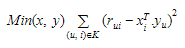Regularization is used to avoid overfitting and generalize the dataset by adding the penalty.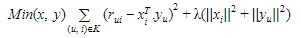#recommendation-system #matrix #singular-values #machine-learning #python1598662200

## The Singularity: When Will We Transition to a Dictatorship of Robots?

Technological Singularity: the moment when robots reach a level of intelligence that is superior to the current level of human reasonableness or ability to predict the outcomes of unrestrained technological growth. As a consequence, the economic, cultural and political singularity of human civilization follows. And although the singularity is still the subject of many Hollywood films and fictions, the possibility of this hypothetical moment genuinely approaching is looking more and more likely.

The process of replacing the biological by technological has already approached the substitution of a human by some algorithm. Thanks to technological acceleration and the accumulation of historical time, the desire to go beyond biology brings mankind closer to the culmination point — the singularity. Acceleration of historical time, that is, the concentration of social events, followed by mankind in a unit of time — is an important factor in the concept of singularity.

The singularity really _is _somewhere near. Moreover, its hypothetical date is real to calculation by scientists, and such data has been presented to the public. After making their calculations, scientists claim to have easily found the starting point of the “singularity”.

It occurs around the middle of the 21st century, which coincides with the forecasts by Ray Kurzweil, who, based on the dynamics of the exponential development of computer technology, predicts that:

• · By 2029 the machine will pass the Turing test;
• · By 2034 artificial intelligence will be created;
• · By the 2040s the non-biological mind will be more powerful than the biological one;
• · By the end of 2040 the Earth will turn into a reasonable “computer”, more precisely, a networked intelligent being;
• · At the end of the century this computer will cover the Universe with its powers

#artificial-intelligence #robotics #machine-learning #future #the-singularity #what-is-the-singularity-theory #hackernoon-top-story #when-will-singularity-happen1624575120

## Java: How to Get Keys and Values from a Map

#### Introduction

Key-value stores are essential and often used, especially in operations that require fast and frequent lookups. They allow an object - the key - to be mapped to another object, the value. This way, the values can easily be retrieved, by looking up the key.

In Java, the most popular `Map` implementation is the `HashMap` class. Aside from key-value mapping, it’s used in code that requires frequest insertions, updates and lookups. The insert and lookup time is a constant O(1).

In this tutorial, we’ll go over how to get the Keys and Values of a map in Java.

#java #java: how to get keys and values from a map #keys #map #values #how to get keys and values from a map